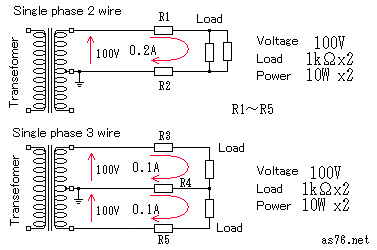﻿

# Single phase two-wire and three-wire system (difference)

In the wiring method from the transformer on a domestic power supply from electric power company, that is, utility pole to each home, it has changed from the single-phase two-wire system to the single-phase three-wire system.

It becomes impossible to correspond by two single phase line type by the consumption of electricity's increasing in a recent home though two single phase line type was natural in an old old house. Then, having appeared is a supply of electric power method of the single-phase three-wire system.

Because the neutral line is grounded, the voltage of ground of the electric wire becomes like 100V though 200V can be used for the single-phase three-wire system. The voltage is high and no translation of danger especially.

Moreover, so in the single-phase three-wire system the IH heater The appliance of 200V with large power such as (electromagnetic cooker) and electric water heaters can be used. Because only half the current flows in 200V with an equipment of the same power consumption compared with the equipment of 100V, the high-power can be handled by even wiring for the same thickness.

Then, the comparison calculation was theoretically done by two single phase line type and the single-phase three-wire system. there was a difference of which extent in the electric power transmission

## Circuit of single phase two-wire and single-phase three-wire systemFigure on this is two single phase line type, and figure below is a schematic diagram of the single-phase three-wire system. I think that it is understood that two single phase line type uses only partially 100 the V of the single-phase three-wire system.

To compare it theoretically, I calculated in the single-phase three-wire system with the current had been balanced.

It is assumed the one to use both of the load resistance of 100V of the two resistance of 1k Ω as a simplified example. This is connected in parallel in two single phase line type. The single-phase three-wire system connects it with 100V for each as shown in figure. Moreover, resistance (R1,R2,R3,R4,R5) of each electric wire is assumed to be 1 Ω by the thickness of the electric wire using all the same things.

Then, the load resistance becomes 500 Ω in two single phase line type, and the current of 0.2A flows. Power consumption becomes 20 a W in total. (Because the power consumption of the electric wire is small, disregard it here. )

The current of 0.1A flows to each resistance in the single-phase three-wire system. Power consumption becomes 10W because of each resistance, and becomes 20 a W in total.

### Comparison of voltage descents with electric wire

The voltage descent with the electric wire of two single phase line type increases to twice that of 0.2x1=0.2V, and becomes 0.4V in total. In a word, the voltage that hangs to the load : It becomes 100-0.4=99.6V.

It is counterbalanced and the current doesn't flow because the current of 0.1A to which the phase shifts by 180 degrees will flow from both direction to R4 when thinking about the voltage descent with the electric wire of the single-phase three-wire system. Therefore, the voltage descent of 100V load becomes 0.1x1=0.1V. In a word, the voltage that hangs to the load becomes 100-0.1=99.9V.

It is understood to become 1/4 in the single-phase three-wire system compared with two single phase line type if the voltage descents with the electric wire are compared. In a word, the single-phase three-wire system is that the voltage descent with the electric wire it becomes 1/4 compared with two single phase line type if it is the same electric wire, and the same electric power.

When the current doesn't flow to R4, and the voltage descent of R3 and R5 is added, it becomes twice at 100V if it thinks about 200V circuit by the single-phase three-wire system. It still becomes 1/4 in the single-phase three-wire system compared with two single phase line type if it thinks at the voltage descent rate because the voltage at this time becomes 200V.

It is necessary to compare the voltage descents here between 100V by the translation to compare the methods of the single-phase three-wire system and two single phase line type.

### Comparison of power consumption with electric wire

Power consumption in the electric wire of two single phase line type increases to twice that of 0.2x0.2=0.04W, and becomes 0.08W in total.

Power consumption in the electric wire of the single-phase three-wire system increases to twice that of 0.1x0.1=0.01W, and becomes 0.02W in total.

It is understood to become 1/4 in the single-phase three-wire system compared with two single phase line type if power consumption in the electric wire is compared. In a word, the single-phase three-wire system is that generation of heat of the electric wire it becomes 1/4 compared with two single phase line type if it is the same electric wire, and the same electric power.

The single-phase three-wire system these are to the last in the state of the ideal, and if the load doesn't do the balance, doesn't become it like this either.

## Notes of type wiring of three single phase lines

• The type wiring of three single phase lines are two system 100V circuit, and should arrange the load thinking about each current balance.
• A voltage that is higher than 100V might hang in each load when the grounded neutral line is disconnected. It is necessary to take care about the thing that there is not loosening of the thing and the connection that doesn't put a fuse in the neutral line especially.
• Or, install the leak breaker with the neutral [senkasou] protection function.